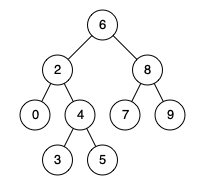Comment on page

# Lowest Common Ancestor of a Binary Search Tree

Given a binary search tree (BST), find the lowest common ancestor (LCA) of two given nodes in the BST.
According to the definition of LCA on Wikipedia: “The lowest common ancestor is defined between two nodes p and q as the lowest node in T that has both p and q as descendants (where we allow a node to be a descendant of itself).”
Given binary search tree: root = [6,2,8,0,4,7,9,null,null,3,5]Example 1:
Input:
root = [6,2,8,0,4,7,9,null,null,3,5], p = 2, q = 8
Output:
6
Explanation:
The LCA of nodes 2 and 8 is 6.
Example 2:
Input:
root = [6,2,8,0,4,7,9,null,null,3,5], p = 2, q = 4
Output:
2
Explanation:
The LCA of nodes 2 and 4 is 2
, since a node can be a descendant of itself according to the LCA definition.
Note:
• All of the nodes' values will be unique.
• p and q are different and both values will exist in the BST.

## Solution & Analysis

### Using attribution of a BST

/**
* Definition for a binary tree node.
* public class TreeNode {
* int val;
* TreeNode left;
* TreeNode right;
* TreeNode(int x) { val = x; }
* }
*/
class Solution {
public TreeNode lowestCommonAncestor(TreeNode root, TreeNode p, TreeNode q) {
// Value of current node or parent node.
int parentVal = root.val;
// Value of p
int pVal = p.val;
// Value of q;
int qVal = q.val;
if (pVal > parentVal && qVal > parentVal) {
// If both p and q are greater than parent
return lowestCommonAncestor(root.right, p, q);
} else if (pVal < parentVal && qVal < parentVal) {
// If both p and q are lesser than parent
return lowestCommonAncestor(root.left, p, q);
} else {
// We have found the split point, i.e. the LCA node.
return root;
}
}
}
Iterative

### Same code as Lowest Common Ancestor of a Binary Tree

public class Solution {
public TreeNode lowestCommonAncestor(TreeNode root, TreeNode p, TreeNode q) {
if(root == null || root == p || root == q) return root;
TreeNode left = lowestCommonAncestor(root.left, p, q);
TreeNode right = lowestCommonAncestor(root.right, p, q);
if(left != null && right != null) return root;
return left != null ? left : right;
}
}